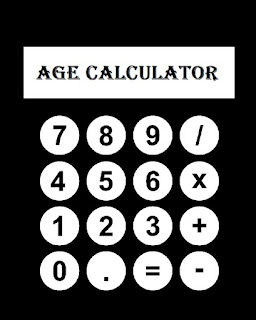# Simple Calculator

0
58CalculateTag- online calculator,simple calculator download,standard calculator,google calculator,simple calculator aws,how to use a basic calculator,percentage calculator,scientific calculator,free calculator

It required for mathematical operations like: calculator for addition, calculator for subtraction, calculator for multiplication, calculator for division. This is a simplified version of the online calculator.

How to use
You can use the [Enter] key to enter the equal character.
To delete the last character, press the Backspace key.
To enter a plus sign, use the [ + ] key at the top or on the side numeric keypad.
To enter a minus sign, use the [ – ] key at the top or on the side numeric keypad.
Use the [ * ] key on the side numeric keypad to enter the multiplication sign.
Use the [ / ] key on the side numeric keypad to enter the division sign.
You can use the [Esc] and [Del] keys on top of the keyboard, or the [End] key on the side numeric keypad to reset the calculator.